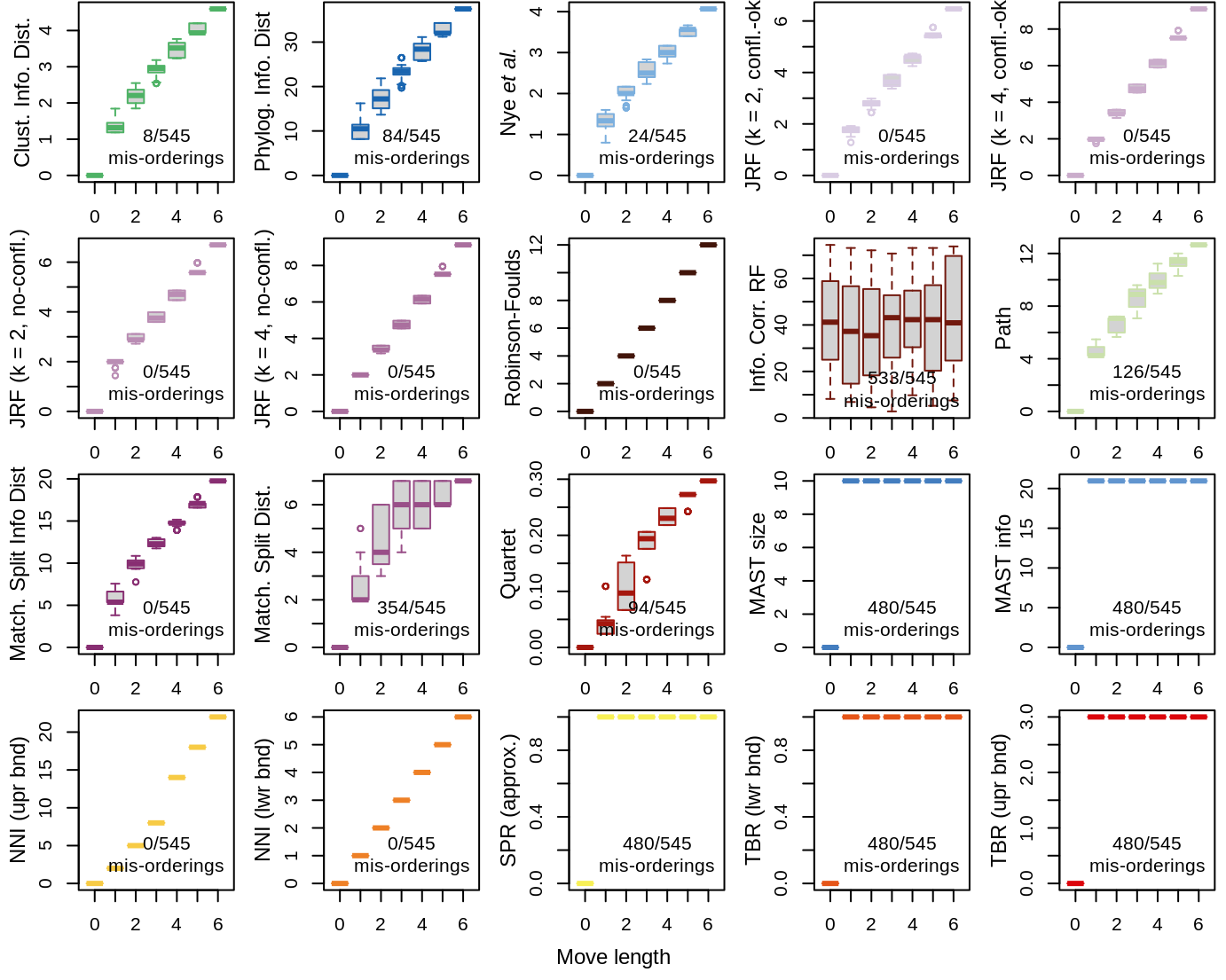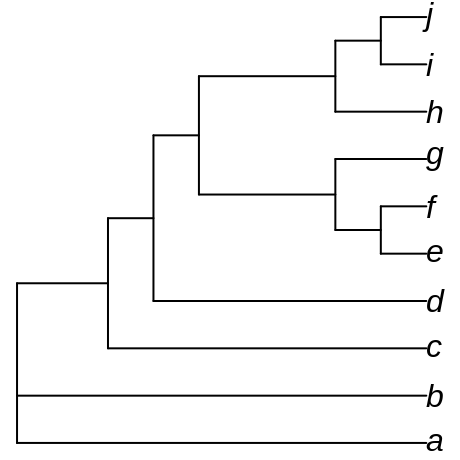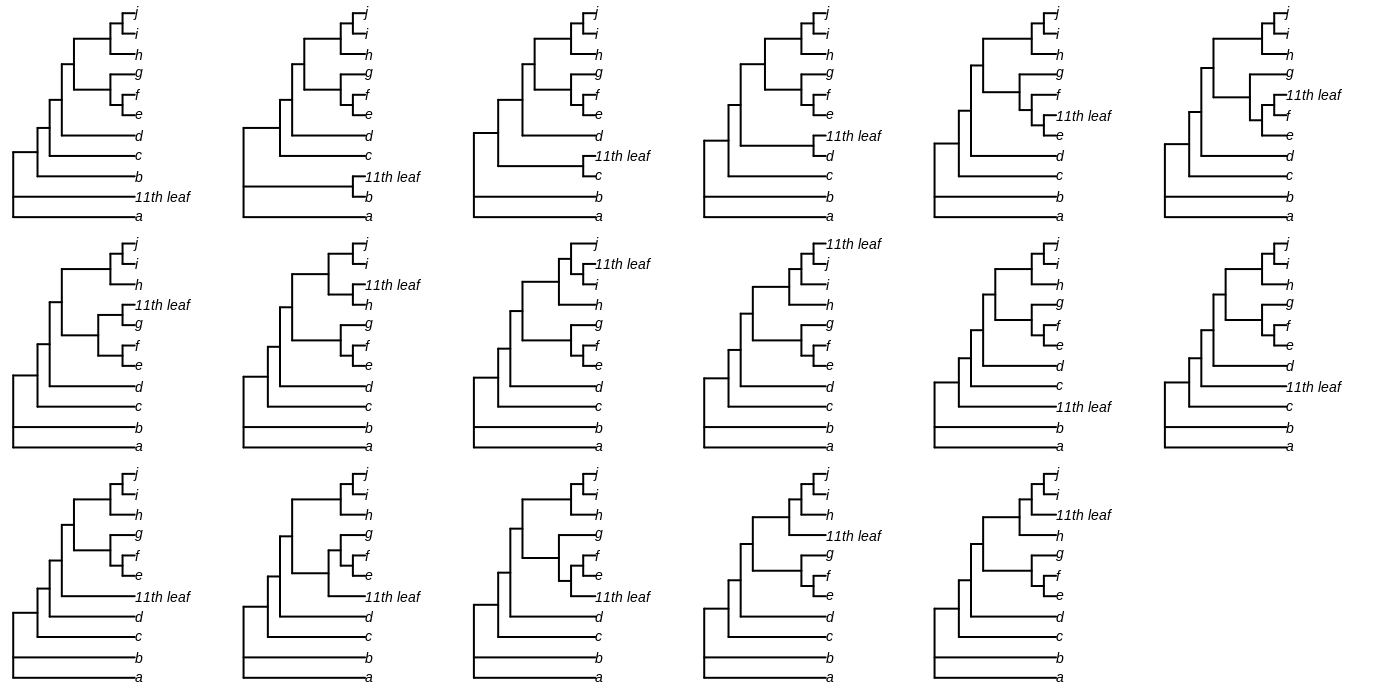## Warning in outer(SplitwiseInfo(tree1), SplitwiseInfo(tree2), "+")[, , drop =
## TRUE] - : longer object length is not a multiple of shorter object lengthDistances between eleven-leaf trees differing in the position of a single leaf. Move length is the number of nodes that must be traversed to travel from the position of the moved leaf in the first tree to its position in the second. If a pair does not have a higher distance than all pairs with a shorter move length, or does not have a lower distance than all pairs with a longer move length, a mis-ordering is recorded.

## Method

I generated seventeen eleven-tip trees that differ only in the position of their eleventh leaf.

These trees were generated by adding an eleventh leaf to each edge in an unrooted unrooted ten-leaf tree:The move length between any pair of these trees is defined as the number of nodes that must be traversed to travel from the position of the eleventh leaf in one tree to its position in the second. I define a mis-ordering as a case where the distance between a pair of trees is not strictly larger than the distance between every tree pair with a shorter move length, or a case where the distance is not strictly smaller than that between every tree pair with a greater move length.

We would like a metric to display a monotonic increase, such that all tree pairs with n edges between the added leaves are further apart than any tree pair with n − 1 edges between the added leaves.